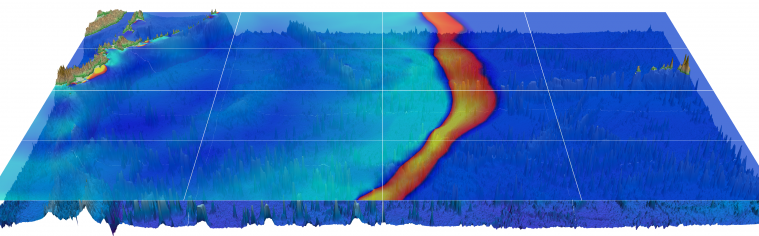# Hyperbolic PDE Seminar, Winter 2019In this seminar we address numerical methods for the simulation of hyperbolic partial differential equations. We discuss important examples of governing equations. In this context challenges typical for hyperbolic PDEs are tackled: Non-linearities with analytical solution approaches, Riemann solvers, domain decomposition, finite volume methods, high-order discretization, time stepping schemes, adaptivity, parallelization etc. Besides numerical theory we expect the students to apply and implement the learned concepts in the form of a small project, which requires extensive use of the learned theory.